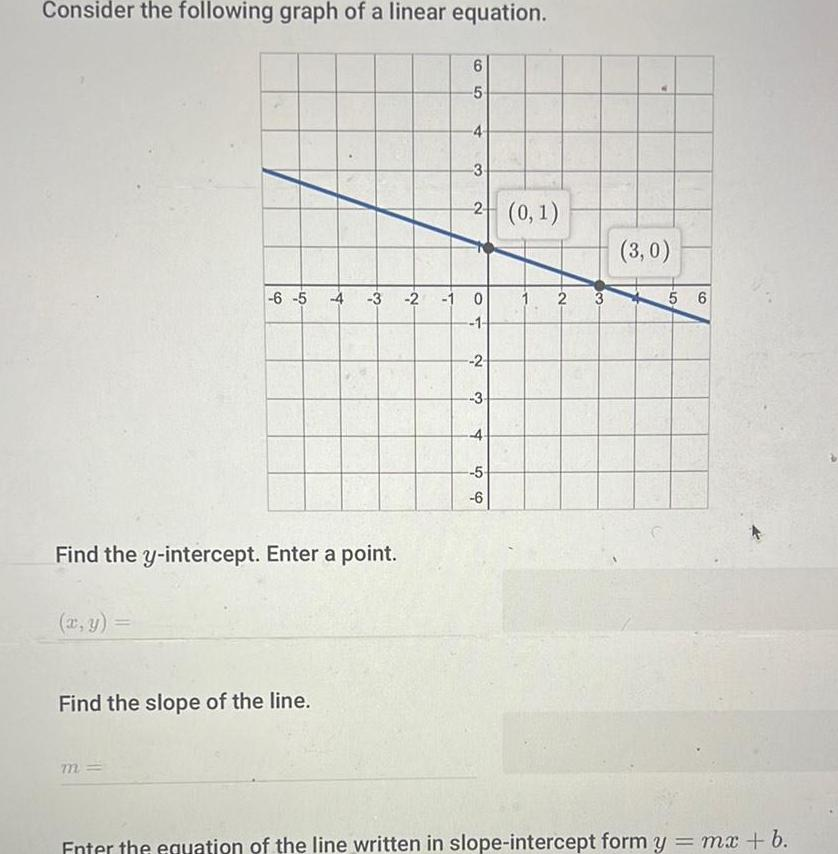Question:

# Consider the following graph of a linear equation 6 5 Find

Last updated: 9/15/2023Consider the following graph of a linear equation 6 5 Find the slope of the line m T Find the y intercept Enter a point 6 5 4 3 2 0 1 3 2 1 0 1 2 3 4 5 6 2 3 3 0 5 6 Enter the equation of the line written in slope intercept form y mx b